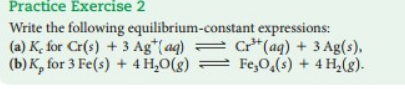# Problem: Write the following equilibrium-constant expressions: (a) Kc for Cr (s) + 3Ag+ (aq) ⇌ Cr3+ (aq) + 3Ag (s), (b) Kp for 3Fe (s) + 4H2O(g) ⇌ Fe3O4 (s) + 4H2 (g).

🤓 Based on our data, we think this question is relevant for Professor Dixon's class at UCF.

###### FREE Expert Solution###### Problem Details

Write the following equilibrium-constant expressions:

(a) Kc for Cr (s) + 3Ag+ (aq) ⇌ Cr3+ (aq) + 3Ag (s),

(b) Kp for 3Fe (s) + 4H2O(g) ⇌ Fe3O(s) + 4H2 (g).